# Sdf3d

3D Signed Distance Field
Alternatives To Sdf3d
3d Machine Learning8,647
4 months ago19
A resource repository for 3D machine learning
Pytorch3d7,34052 days ago10April 28, 2022187otherPython
PyTorch3D is FAIR's library of reusable components for deep learning with 3D data
Draco5,664132017 days ago23July 07, 2022100apache-2.0C++
Draco is a library for compressing and decompressing 3D geometric meshes and point clouds. It is intended to improve the storage and transmission of 3D graphics.
Meshlab3,671
2 months ago141gpl-3.0C++
The open source mesh processing system
Cloudcompare2,722
5 days ago186otherC++
CloudCompare main repository
Graphics2,70831a month ago25December 03, 2021147apache-2.0Python
TensorFlow Graphics: Differentiable Graphics Layers for TensorFlow
Yocto Gl2,590
a month ago9C++
Yocto/GL: Tiny C++ Libraries for Data-Driven Physically-based Graphics
Pyvista1,810139920 hours ago60June 30, 2022354mitPython
3D plotting and mesh analysis through a streamlined interface for the Visualization Toolkit (VTK)
Online3dviewer1,646
a day ago10June 13, 202279mitJavaScript
A solution to visualize and explore 3D models in your browser.
Vedo1,63912a day ago18May 08, 202292mitPython
A python module for scientific analysis of 3D data based on VTK and Numpy
Alternatives To Sdf3d
Select To Compare

Alternative Project Comparisons

# Signed distance field (SDF) in 3D

[Fuhrmann,2003] introduces an efficient method to calculate the SDF of 3D objects.

In their paper, they state that the distance from a point to a triangle can be calculated using Voronoi regions, but they do not provide any details. After a lot of searching, I found this article written by Erin Catto which explains exactly how to calculate that distance using Voronoi regions.

Strongly recommend you to use SDFGen from Christopher Batty. It is much more efficient and accurate than mine.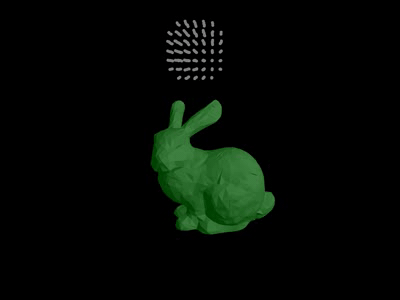Permission is hereby granted, free of charge, to any person obtaining a copy of this software and associated documentation files (the "Software"), to deal in the Software without restriction, including without limitation the rights to use, copy, modify, merge, publish, distribute, sublicense, and/or sell copies of the Software, and to permit persons to whom the Software is furnished to do so, subject to the following conditions:

The above copyright notice and this permission notice shall be included in all copies or substantial portions of the Software.

THE SOFTWARE IS PROVIDED "AS IS", WITHOUT WARRANTY OF ANY KIND, EXPRESS OR IMPLIED, INCLUDING BUT NOT LIMITED TO THE WARRANTIES OF MERCHANTABILITY, FITNESS FOR A PARTICULAR PURPOSE AND NONINFRINGEMENT. IN NO EVENT SHALL THE AUTHORS OR COPYRIGHT HOLDERS BE LIABLE FOR ANY CLAIM, DAMAGES OR OTHER LIABILITY, WHETHER IN AN ACTION OF CONTRACT, TORT OR OTHERWISE, ARISING FROM, OUT OF OR IN CONNECTION WITH THE SOFTWARE OR THE USE OR OTHER DEALINGS IN THE SOFTWARE.

# Find the distance from a point to a triangle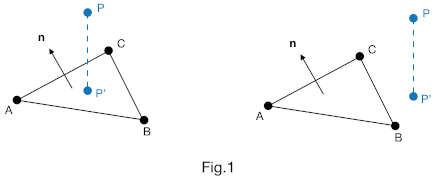For example, in Fig.1, we want to find the distance from `P` to `ΔABC`. We first project `P` onto the plane of `ΔABC` to get `P'`. By examining the barycentric coordinate of `P` with respect to `ΔABC`, we can know whether `P'` is inside `ΔABC` or not. If inside, then `dist = dot(n, AP) * theSignOf(dot(n, AP))`.

Otherwise, we must find the closest edge (Fig.2 left) or vertex (Fig.2 right) of `ΔABC` to `P`.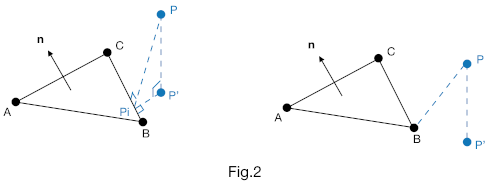One way to achieve this is to use Voronoi regions. Details of the algorithm can be found in Erin Catto's article. Here, I only provide the summary (Fig.3).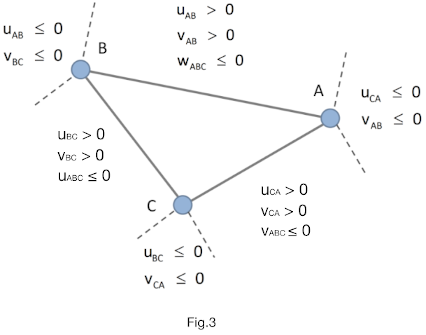Note that the `(u,v)` of a line segment and the `(u,v,w)` of a barycentric coordinate may be different due to the definition we use. In that case, the conditional statements in Fig.3 may also be different. So be careful with that.

# The barycentric coordinates system

The full article of calculating the barycentric coordinate of a point can be found here.

For short, if a triangle is defined by vertices `ABC` in counter-clockwise, and `P` is a point on the same plane with `ABC`, then `P` can be described as `P = uA + vB + wC`. Here, `(u, v, w)` is called the barycentric coordinate of `P` with respect to `ABC`.

`(u, v, w)` can be calculated using the ratio of triangle areas.

Let `Sabc`, `Sabp`, `Sbcp` and `Scap` be the area of triangle `ABC`, `ABP`, `BCP` and `ACP`, respectively. Then `(u, v, w)` can be calculated as

``````u = Sbcp/Sabc
v = Scap/Sabc
w = Sabp/Sabc
``````

## The signed area of a triangle

If a triangle is defined by vertices `ABC`, then its area can be calculated as `Sabc = 0.5 * length(cross(AB, AC))`.

Note that the magnitude of the cross product of `AB` and `AC` defines the area of a parallelogram, and the area of a triangle is equal to half of that area, i.e.

``````Sparallelogram = |AB|*|AC|*sin(∠A) = length(cross(AB, AC))
Striangle = 0.5 * Sparallelogram
``````

Be careful that the order of the vectors in cross product matters. For example, when point `P` is outside the triangle, the four areas will have different signs. In this case, we must use something like

``````vec3 temp = cross(AB, AC);
float area = 0.5 * length(temp) * sign;
``````

to calculate the signed area.

In 2D, we can use the z-component to decide `sign`, i.e.

``````float sign = (temp.z > 0) ? 1 : -1;
``````

In 3D, However, things become a little different because `temp` can points to any direction. As a result, we cannot decide `sign` only based on z-component.

Instead, we can use the surface normal `N` of `ΔABC`. If `temp` points to the same direction as `N` does, then we decide `sign = 1`. Otherwise, `sign = -1`, i.e.

``````float sign = dot(normalize(temp), N);
``````

# Special case

This case is essentially equal to the "changing signs" cases pointed out by [Fuhrmann, 2003].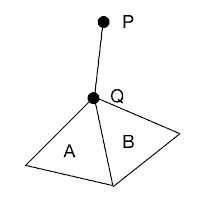Assume that there are two adjacent triangles `A` and `B`, and point `Q` is one of their shared vertices. `P` is a point outside the triangle, and the distance from `P` to `A` and `B` are both `PQ`. `P` is forward to `B`, but is backward to `A`. So the corresponding signed distance are `|PQ|` and `-|PQ|`, respectively.

In this case, `P` is obviously outside the mesh. However, if we simply use `abs(newDist) < abs(oldDist)` to decide the new distance, errors happen. That is, if `B` is previously iterated than `A`, then the signed distance will always be `-|PQ|`, i.e. `P` will be decided as inside the mesh.

To solve this problem, we assume that if `abs(newDist) == abs(oldDist)`, but their signs are different, we keep the positive one as the new distance. This correctly decides `P` as outside the mesh.

# Result

## SDF-based collision detection

Add some simple simulation code to test the SDF-based collision detection.

To increase accuracy, we can use smaller `cellSize` when creating SDF, and smaller threshold when doing collision detection.

## Use sdf3d as a solid voxelizer

A common way to solid-voxelize a mesh is to use octree.

A signed distance field can also be used as a solid voxelizer.

``````for the entire field:
if(signedDistance < 0) voxelPosition = cellPosition;
``````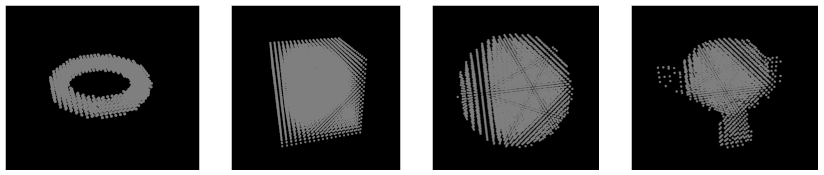# Note

When calculating SDF for a mesh, we use surface normals, not vertex normals. So be careful when you export your mesh data from your 3D modeling software (e.g. Blender). Especially, if you use a smooth shader, you should prepare two same meshes. One with surface normals for calculating SDF, the other one with vertex normals for smooth shading.

# Reference

[Fuhrmann,2003] Fuhrmann, Arnulph, Gerrit Sobotka, and Clemens Groß. "Distance fields for rapid collision detection in physically based modeling." Proceedings of GraphiCon 2003. 2003.

Popular 3d Graphics Projects
Popular Mesh Projects
Popular Graphics Categories
Related Searches

Get A Weekly Email With Trending Projects For These Categories
No Spam. Unsubscribe easily at any time.
C Plus Plus
3d Graphics
Mesh
Collision Detection